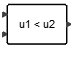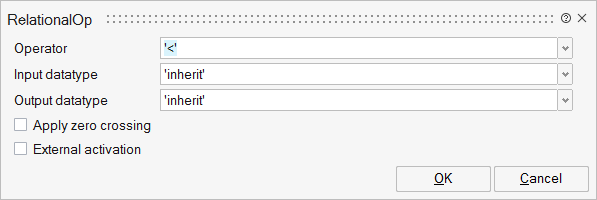# RelationalOp

This block compares two input signals defined by the relational operator (parameter).## Library

LogicalOperations

## Description

The RelationalOp block compares two input signals defined by the relational operator (parameter).

Supported relational operations are : "==", "~=", "<", "<=", ">" and ">=".

if the operator is "==", the result is TRUE if the two inputs are equal.

if the operator is "~=", the result is TRUE if the two inputs are not equal.

if the operator is "<", the result is TRUE if the first input is less than the second input.

if the operator is "<=", the result is TRUE if the first input is less or equal than the second input.

if the operator is ">", the result is TRUE if the first input is greater than the second input.

if the operator is ">=", the result is TRUE if the first input is greater or equal than the second input.

## ParametersNameLabelDescriptionData TypeValid Values

rule

Operator

Relational operations supported by the RelationalOp block are : "==", "~=", "<", "<=", ">" and ">=".

String

'=='
'~='
'<'
'<='
'>'
'>='

typei

Input datatype

Defines the datatype of the inputs.
Supported types are : double, int32, int16, int8, uint32, uint16, uint8, boolean, inherit.

String

'double'
'complex'
'int32'
'int16'
'int8'
'uint32'
'uint16'
'uint8'
'boolean'
'inherit'

typeo

Output datatype

Defines the datatype of the output.
Supported types are : double, int32, int16, int8, uint32, uint16, uint8, boolean, inherit.

String

'double'
'complex'
'int32'
'int16'
'int8'
'uint32'
'uint16'
'uint8'
'boolean'
'inherit'

zcr

Apply zero crossing

Parameter to activate zero-crossing detection.

Scalar

0
1

externalActivation

External activation

This parameter defines if the block receives an external activation or inherits. When External Activation is used, an activation port is added to the block.

Number

0
1

## Ports

NameTypeDescriptionIO TypeNumber

Port 1

explicit

input

1

Port 2

explicit

input

2

Port 3

explicit

output

1

Port 4

activation

input

externalActivation

NameValueDescription

always active

no

direct-feedthrough

yes

zero crossing

no

mode

no

continuous-time state

no

discrete-time state

no